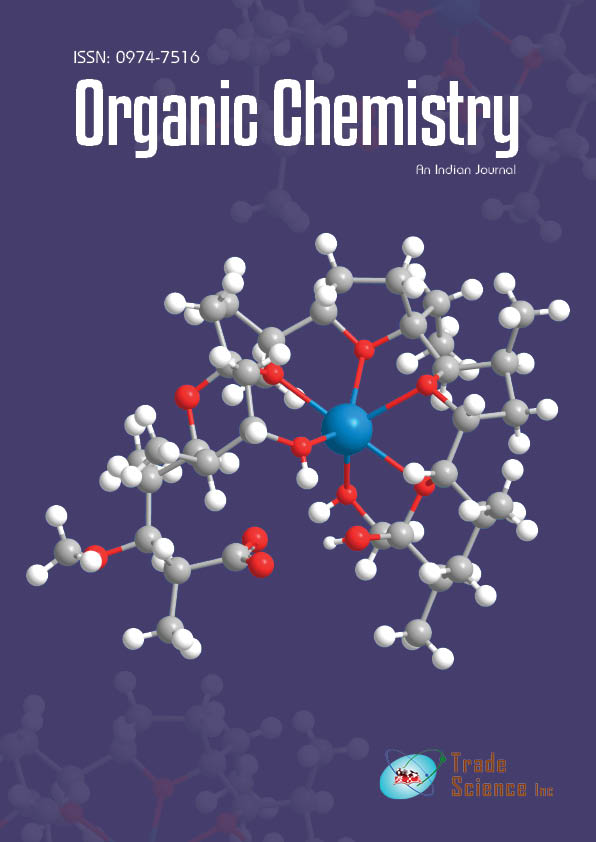All submissions of the EM system will be redirected to Online Manuscript Submission System. Authors are requested to submit articles directly to Online Manuscript Submission System of respective journal.

# Original Article

, Volume: 14( 2)

## Computational Prediction of Synthetic Accessibility of Organic Molecules with Ambit-Synthetic Accessibility Tool

*Correspondence:
Nikolay Kochev, Faculty of Chemistry, University of Plovidv “P. Hilendarski”, 24 Tsar Assen str., Plovdiv 4000, Bulgaria, Tel: (032) 602-507; E-mail: [email protected]

Received: April 28, 2018; Accepted: May 5, 2018; Published: May 22, 2018

Citation: Kochev N, Avramova S, Angelov P, et al. Computational Prediction of Synthetic Accessibility of Organic Molecules with Ambit-Synthetic Accessibility Tool. Org Chem Ind J. 2018;14(2):123

### Abstract

The wide use of computer assisted synthesis design nowadays as well as many newly developed de novo design methods applied to large chemical structure databases necessitate subsequent synthesis of the compounds. In addition to the desired physicochemical properties and biological activities, the target compounds must be synthetically accessible under real laboratory conditions. In this context we present a model for computational prediction of the synthetic accessibility (SA) using four weighted molecular descriptors, representing different structural and topological features, combined by an additive scheme. The components of SA modeling function are examined and the results from the comparison of our model with data from other methods are presented. The method for theoretical prediction of SA is implemented within open source software tool Ambit-SyntheticAccessibility (Ambit-SA).

### Keywords

Synthetic accessibility; Molecular complexity; Computer modeling; Aadditive scheme

### Introduction

Synthesis of some compounds under laboratory conditions appears to be extremely difficult. In this context, the synthetic accessibility (SA), represented as a formal property (or molecular descriptor), plays even a more important role than many chemoinformatics descriptors and medicinal chemistry parameters obtained by QSAR modelling. While the biological activity or the toxicity can be measured experimentally, synthetic accessibility (SA) is a more abstract and cannot be easily assessed . The software models for the evaluation of synthetic accessibility (SA) or facilitating the synthesis are created in order to assess large number of potential drug-like candidates, generated by combinatorial libraries or de novo molecular design methods .

Software systems for synthetic accessibility prediction can be classified into three main categories: based on complexity, based on starting materials and based on retrosynthetic analysis.

Methods based on starting materials evaluate SA by determining how much of the target compound overlap with the available starting materials. To identify possible starting materials, one of the following two approaches is commonly used: methods based on the exact match of substructures and methods based on similarity; either on substructures or on the target structure . Since the use of chemical knowledge in these methods is limited, small differences in structures and functional groups may lead to a significant difference in synthetic accessibility . Fukunishi et al.  use a compound library to evaluate the probability of the existence of substructures contained within the target compound as well as information about symmetry atoms, graph complexity and chiral centers. Daina et al.  analyze more than 13 million compounds available on the market to identify the most common molecular fragments, suggesting that the frequent occurrence of certain molecular fragments is an indication of high synthetic accessibility and the rare fragments are difficult to synthesize. Schürer et al.  offer a new approach to exploring a synthetically feasible chemical space of small molecules of commercially available starting materials by applying computational QSAR models and a series of physicochemical and structural filters.

Complexity based analysis is perhaps the most widely used technique in assessing synthetic accessibility because it is effective and easy to implement. One of the earliest molecular complexity calculation methods is proposed by Bertz . He applies concepts from information theory and representation of the molecule structure as a graph characterized by its invariants (e.g. atom classes, bond classes, classes of paths in the graph etc.) which are used to calculate the Shannon entropy (i.e. the molecular complexity). Whitlock  defines a simple metric based on the number of cycles, heteroatoms and chiral centers. The Barone et al.  indexes consider only the relationships of the atoms in the molecule and the size of the cycles. Allu et al.  develop a method based on relative atomic electronegativities and bond parameters.

All the methods for calculating molecular complexity are very fast because no substructure search is needed. However, this is also a disadvantage of these methods, since the complexity by itself is not a sufficient criterion for synthetic accessibility-there are many starting materials of high molecular complexity that are readily available. One of the new approaches based on molecular complexity  attempts to overcome these drawbacks by including a statistical distribution of different cyclic and acyclic topologies and models for replacing atoms in existing commercially available starting materials. The method is based on the assumption that if the molecule contains only structural motifs that are common in commercially available starting materials, it is probably easy to synthesize.

While complexity-based approaches are fast enough for primary screening, they have some limitations, such as excluding complex molecules that can actually be synthesized by existing complex precursors . To overcome this problem, Ertl proposes a SA predictive model  based on equation (1) the frequency of occurrence of molecular fragments  in a compound database (PubChem) and (2) assessing the complexity of the molecule..

Compared to methods based on complexity and starting materials, retrosynthetic analysis based assessment methods use a wider range of chemical knowledge; according to medical chemists, this is the most acceptable approach . RASA (Retrosynthesis-Based Assessment of Synthetic Accessibility)  is a retrosynthesis based method for assessing of synthetic accessibility which takes into account weighted number of possible effective synthetic routes, the complexity of the most favorable reaction and the difficulty of the separation and purification associated with the most favorable synthetic reaction. Another program, CAESA [16,17] also applies retrosynthetic transforms to the target compound and the precursors obtained until a suitable starting material is found. Voršilák et al.  use molecular morphing algorithms in Nonpher-a computational method for constructing a virtual library with compounds that are difficult to synthesize. However, almost all SA evaluation programs based on retrosynthetic analysis are highly interactive and relatively slow, often taking a few minutes to analyze a single structure. This makes these methods unusable for quickly assessing the SA of thousands (or even millions) of molecules, as is usually the case for de novo generation of ligands, for example. To tackle this problem Podolyan et al.  present two approaches for rapid prediction of the synthetic accessibility by using support vector machines working with molecular descriptors.

Some methods combine several of the approaches used to assessing synthetic accessibility. SYLVIA  is a software product developed for Molecular Networks by Boda et al.  which evaluates the synthetic accessibility on the basis of several components: structural complexity, similarity to available starting materials and assessment of strategic bonds where a structure can be decomposed to obtain simpler fragments [20,21]. The SA result is obtained through an additive scheme with weighted components.

### Methodology

Synthetic accessibility prediction algorithm

We present a method for theoretical prediction of synthetic accessibility represented as a score (numerical value), SA, normalized in the range of 0 to 100. Score SA=100 means most easily synthesizable (maximal synthetic accessibility). Score SA=0 (or even negative values in some cases) means very difficult to synthesize or not synthesizable at all (minimal synthetic accessibility). Synthetic accessibility score is calculated on the base of four weighted molecular descriptors scores: Ring Complexity (SRC), Cyclomatic number (Sμ), Stereochemical Complexity (SWSC) and Molecular Complexity (SCM):(1)

The approach described in equation (1) uses modified versions of the structure based components of additive SA model published by Boda et al. . We developed a chemoinformatics algorithms to calculate the SA value of each component: the score for ring complexity of the molecule (SRC) is based on the smallest set of smallest rings (SSSR); the second component is the quite simplistic approach for estimating overall ring number by the cyclomatic number μ; the third component gives information about stereochemical complexity based on the weighted number of chiral centers in the molecule (SWSC). The fourth algorithm calculates molecular complexity (SCM) based on information theory and the examination of all paths in the molecular graph up to a given length.

To convert a given molecular descriptor di into a score (ranging from 0 to 100) for the SA additive scheme, a linear transformation function ki*di+mi is used.

Ring complexity

The ring complexity is considered to be an important part in assessing synthetic accessibility as it is indicative of the presence of bridged and fused systems that could give rise to difficulties in compound synthesis . For calculating the ring complexity of a molecule (RC), we have applied the approach described by Gasteiger et al.  using the following formula: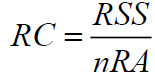(2)

Where, RSS (Ring Size Sum) is sum of the sizes of all rings from SSSR (smallest set of smallest ring) and nRA is the number of all atoms in the molecule involved in at least one cycle. To find the SSSR set, we used the algorithm implemented in CDK library.

RC is normalized in percent value from 0 to 100 by the following formula:(3)

Where, the conversion factors are respectively: kRC=-100, mRC=200. RC value typically is between 1 and 1.5, normalized in the range from 100 to 0%, respectively. A higher ring complexity value (RC) implies a lower contribution to overall synthetic accessibility, as evidenced by negative value of coefficient kRC. If the molecule possesses extreme ring complexity (RC>1.5 very rare), negative SRC values can be obtained as an additional “penalty” contribution to overall SA score.

Cyclomatic number

The cyclomatic number is a value that represents the minimum number of bonds to be disconnected in order to obtain an acyclic graph from a cyclic one. For each graph, it is less than or equal to the number of cycles in the graph. The cyclomatic number as an element in the SA score function is calculated by the formula:(4)

Where, m is the number of bonds and n is the number of atoms of the molecule.

The score for SA function is given by: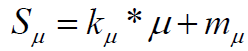(5)

With coefficients: defining a "penalty" function of-10% for each cycle in the structure is introduced by us to be a correction factor to the score component SRC which does not penalize non fused rings at all.

Stereochemical complexity

For assessing of SA, one of the factors to be taken into account is the number of chiral centers. Compounds with one or more stereo centers would impede synthesis; there are also many cases where a molecule has to be synthesized in a precisely defined conformation, making synthesis even more difficult.

To evaluate stereo chemical complexity, we have used the approach described by Cahn et al.  modified by us with a stereo center weighting schema. All stereo elements of the molecule are identified and the stereo chemical complexity score is calculated by the following formula:(6)

Where, NWSC is the number of weighted chiral centers in the molecule, where chiral atoms are given weight 1.0 while stereo double bonds have weight 0.3. Score SWSC is obtained with linear transformation coefficients. A larger number of stereo centers correspond to a lower contribution to the synthetic accessibility of the molecule; thus coefficients define a penalty function of -30% for each chiral center.

Molecular complexity

The calculation of the molecular complexity is based on the fact that molecules can be represented as graphs. The complexity of a molecule is obtained by means of information theory and factorization of the molecule graph into classes of equivalent paths of particular length used to calculate Shannon entropy.

To calculate the complexity of atoms (CA), as well as molecular complexity (CM), we implemented the method described by Proudfoot et al. . Each atom complexity (CA) is calculated as Shannon entropy:(7)

Where, for a given atom, pi=Ni/N is the relative number of the paths of class i starting from this atom and N is the total number of the paths starting from this atom.

The sum of all atoms complexities gives the overall molecular complexity (CM):(8)

The molecular complexity score is normalized using following formula: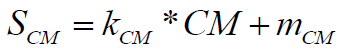(9)

Where, the coefficients have default values, normalizing the molecular complexity of 150 to 0% score and, respectively, molecular complexity 0 to 100% score.

FIG. 1 illustrates the calculation of SA for a complex organic molecule.

RC=27/23=1.174, SRC=82.609=5,=50.0

WSC=2.0, SWSC=40.0

CM=88.751, SCM=40.833

wRC=0.3, wμ=0.1, wWSC=0.2, wCM=0.4

SA=0.3*82.609+0.1*50.0+0.2*40.0+0.4*40.833=54.116

Software implementation

The described above SA estimation method is implemented as a command line interface application Synthetic AccessibilityCli (designated as Ambit-SA). Ambit-SA is developed within ambit2-reactions software module, part of Ambit chemoinformatics platform [25-27]. Ambit-SA is an open source program under LGPL license and can be used freely for academic, regulatory and commercial purposes. An executable jar file can be downloaded from following web address:

http://ambit.sourceforge.net/reactor.html

Ambit-SA can be started from command line by following command:

java -jar SyntheticAccessibilityCli.jar option1 option2 …

SA calculation for a single molecule can be performed by directly entering the molecule SMILES from the command line with option ‘-s’ e.g.

java -jar SyntheticAccessibilityCli.jar -s.

"FC(F)(F)c1cc(ccc1)N5CCN(CCc2nnc3[[email protected]]4CCC[[email protected]]4Cn23)CC5"

Calculating SA for: FC(F)(F)c1cc(ccc1)N5CCN(CCc2nnc3[[email protected]]4CCC[[email protected]]4Cn23)CC5 SA=54.116

Option ‘-v’ can be used for a verbose output:

java -jar SyntheticAccessibilityCli.jar -s

"FC(F)(F)c1cc(ccc1)N5CCN(CCc2nnc3[[email protected]]4CCC[[email protected]]4Cn23)CC5" -v

Calculating SA for: FC(F)(F)c1cc(ccc1)N5CCN(CCc2nnc3[[email protected]]4CCC[[email protected]]4Cn23)CC5

SA=54.116

SA details:

MOL_COMPLEXITY_01 88.751 score=40.833

WEIGHTED_NUMBER_OF_STEREO_ELEMENTS 2.000 score=40.000

CYCLOMATIC_NUMBER 5.000 score=50.000

RING_COMPLEXITY 1.174 score=82.609

Option ‘-i’ can be used to set an input file with a set of structures for a batch calculation:

java -jar SyntheticAccessibilityCli.jar -i sa-mol-set-01-b.smi

Calculating SA for molecule set: sa-mol-set-01-b.smi

# smiles NumAtoms SA

1 CCOP(=S)(OCC)Oc1cc(C)nc(n1)N(C)C 19 85.622

2 OOC1CCOP(=O)(N1)N(CCCl)CCCl 16 81.760

3 O=C1Cc2c(N1)ccc3OCC(CNCc4ccccc4)Oc23 23 64.931

4 CC1OC(=NC1CCOc2ccc(CC3C(=O)NOC3=O)cc2)c4ccccc4 29 72.725

5 O=C(NN1CCCCC1)c2nn(-c3ccc(Cl)cc3Cl)c(-c4ccc(Cl)cc4)c2C 30 72.241

6 CC(C)C(=O)Oc1ccc2CC(CCc2c1OC(=O)C(C)C)NC 24 66.674

### Results and Discussion

The main problem in developing methods for assessing synthetic accessibility is the validation of results . Although quantitative criteria such as yield and number of steps in a synthetic sequence of reactions give some information, the synthetic accessibility depends on many other factors, such as the optimization of the synthetic routes. In this regard, in order to evaluate the effectiveness of our algorithm, we have used track of record for compounds with synthetic accessibility, determined by experts .

Evaluation of the scoring function components

For each molecule of our test set, the scores of the individual components (molecular complexity, stereochemical complexity, cyclomatic number and ring complexity) in the final synthetic accessibility score were calculated.

The data are summarized in TABLE 1, which provides information on Pearson correlation coefficients r between the scores of the individual components and the synthetic accessibility values estimated by medicinal chemists for a set of 40 molecules described in literature .

Score R
SCM 0.867
0.675
SRC 0.565

TABLE 1. Pearson correlation coefficient between SA estimated by medicinal chemists and different SA components of our approach.

There is a high correlation between the calculated molecular complexity and the data given by the chemists. This can be explained by the fact that, on the one hand, the model uses a quantitative assessment of the information content (molecular complexity) based on the molecule topology and, on the other hand, the experts make assessment in a similar fashion based on their own knowledge and experience and in principle large and complex molecules containing condensed or bridged systems are intuitively evaluated as difficult to synthesize.

The moderate correlation of the cyclomatic number score to the chemist's data is expected as it is intended as a correction factor for RC descriptor. Most probably it is due to the fact that much of the test set is represented by compounds that do not contain fused cycles (which could be a possible reason for higher values for the cyclomatic number).

Pearson's correlation coefficient is the lowest among the data on synthetic accessibility given by chemists and the ring complexity score. However value r=0.565 is a good enough correlation between single descriptor and an estimated property. We consider this component as important part of the model for synthetic accessibility evaluation.

With regard to the stereo chemical complexity score, it should be noted that compounds with stereo centers were not found in the set of compounds for which data on synthetic chemistry accessibility were available. In this regard, the contribution of the stereochemical complexity of the compounds has been evaluated on the basis of literature data .

Evaluation of calculated synthetic accessibility

The optimal weight values (wRC, wμ, wWSC and wCM) were determined by a gird search where the goal was maximal correlation coefficient between SA model and the chemical expert scores. The optimal values for weights are shown in TABLE 2.

wi Weight
wMC 0.4
wWSC 0.2
0.1
wRC 0.3

TABLE 2. Optimal weights of Ambit-SA model components.

The optimal weight values (wRC, wμ, wWSC and wCM) were determined by a gird search where the goal was maximal correlation coefficient between SA model and the chemical expert scores. The optimal values for weights are shown in TABLE 2.

Synthetic accessibility data from the literature is given as numbers ranging from 0 (most accessible molecules) to 10 (for the hardest to synthesize) which, in order to be compared with our results, were converted by linear transformation to the scoring scheme implemented in Ambit-SA.

TABLE 3 contains the SA results and the structures for which there is a significant difference between the results of our model and the values given by chemists:

The higher values for the synthetic accessibility given by Ambit-SA compared to the values given by medical chemists for all structures in TABLE 3 can be explained by the fact that our model (Ambit-SA) considers the topology of the molecule but does not take into account the possibilities for its synthesis (in the additive scheme of Ambit-SA there is no component based on retrosynthetic analysis).

Structure SA
(Ambit-SA)
SA
(medicinal chemists)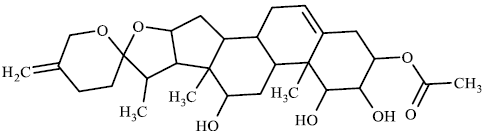49.46 18.950.26 34.453.35 23.3

TABLE 3. Comparison between synthetic accessibility calculated by Ambit SA and results provided by medicinal chemists.

Comparison to other models

The synthetic accessibility data calculated by Ambit-SA are compared with the results published by Ertl et al. , whose model uses an approach based on complexity of molecules and the frequencies of typical fragments found in the commercial collections of staring materials. The Pearson correlation coefficient between the two models is 0.862 (TABLE 4). The authors consider that the fragmentation of the molecules, used in their synthetic accessibility model, bear a resemblance to the methods using similarity to starting materials. Given that our model does not take into account similarity to starting materials, the high correlation of our results with the Ertl’s model is an indication that Ambit-SA is reliable enough and includes components based on structural information that are relevant to assessing synthetic accessibility.

Model Number of compounds used Correlation with Ambit-SA (r)
ERTL 40 0.862
SYLVIA 40 0.938
FA4 130 0.634

TABLE 4. Pearson correlation coefficient between Ambit-SA and other software tools.

The results obtained from Ambit-SA were compared with the SA model data for same compounds calculated by SYLVIA  (TABLE 4). The high correlation coefficient (0.938) is expected as long as both methods are similar and combine molecular complexity assessment, chiral center counts and ring complexity in their algorithms. In both models, the molecular complexity score in the calculation of synthetic accessibility has a high weight-this can be considered as a reason for quite close final results calculated by SYLVIA and Ambit-SA. Unlike Ambit-SA, however, SYLVIA uses another component that have their contribution to the synthetic accessibility score: similarity to starting materials and retrosynthetic fitness.

Ambit-SA software tool was compared with a model (FA4) based on the starting materials . For 130 compounds taken from the literature , the correlation obtained between the two methods is 0.634. The latter result is also expected, since the FA4 algorithm is based on a completely different type of chemical information i.e. the similarity of target molecule to available starting materials.

The comparison between different SA models and SA values estimated by the chemist-experts is quite challenging on the one hand and the other hand SA score is a formal chemical property expressed as a single value descriptor which is impossible to encompass the entire chemical logic and corresponding synthetic context. Having in mind the principle drawbacks of the SA concept, the utilization of SA models is efficient especially in context of handling large chemical structure sets for a fast screening of molecule candidates.

### Conclusion

The synthetic accessibility predictive model implemented in Ambit-SA tool can be employed as a useful tool for assessing the ability of organic compounds to be synthesized under real laboratory conditions. The results of the comparison of Ambit-SA with other data obtained from experts and models based on molecular complexity, starting materials and combined approaches are indicative to the effectiveness of Ambit-SA tool. Additionally, the components of additive scheme can be configured by the user with weights that may fit particular objectives. Another advantage of our algorithm is that it is fast and can be used in a batch mode, which is crucial when working with a large number of compounds (e.g. combinatorial libraries). Ambit-SA (http://ambit.sourceforge.net/reactor.html) is an open source software tool with LGPL license, part of the dynamically developed chemoinformatics platform Ambit and can be used for academic, regulatory and commercial purposes. The planned future development of Ambit-SA includes improvement of ring complexity score as well as handling of additional components of synthetic accessibility such as similarity to starting materials and retrosynthetic paths contribution that will account for two very important aspects of the synthetic logic. The similarity to starting materials will be handled on the base of recognizing starting material fragments and estimation of the degree of overlapping between these fragments and the target molecule. A retrosynthetic based score is also envisaged to be included in Ambit-SA. We have already developed a software module for computer based retrosynthetic analysis which will be used for counting and scoring the efficiency of the generated retrosynthetic paths as long as we consider it as an important element of SA estimation.

### References

Select your language of interest to view the total content in your interested language

###### Citations : 521

Organic Chemistry: An Indian Journal received 521 citations as per Google Scholar report

#### Indexed In

• CASS
• Open J Gate
• China National Knowledge Infrastructure (CNKI)
• Cosmos IF
• Directory of Research Journal Indexing (DRJI)
• Secret Search Engine Labs